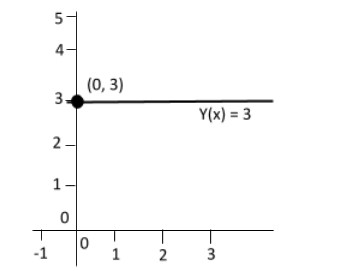QuestionAnswers

# Define constant function. Also write its graph. Domain and range of function.Verified
128.7k+ views
Hint: A function is a rule which relates the values of one variable quantity to the values of another variable quantity.

Complete step by step solution: A constant function is a function whose (output) value is the same for every input value.
For example, the function $y\left( x \right) = {\text{3}}$ is a constant function because the value of $y\left( x \right)$ is ${\text{3}}$ regardless of the input value $x$.
Domain of function: The domain of a function is the complete set of possible values of the independent variable.
If$f\left( x \right) = c$, they consist of all real numbers, there are no restrictions on the input. The only output value is the constant${\text{c}}$, so the range of the set $\left\{ c \right\}$ that contains this single elementRange function: The range of a function is the complete set of all possible resulting values of the dependent variable of
For example:
Here: It is a set in the form of (${\text{x,y}}$): $\left\{ {( - 3,5),( - 2,5)( - 1,5)(2,5)(1,5)(2,5)} \right\}$
The values of$\;{\text{y -}}$values for the range.
Then range $= \left\{ 5 \right\}$

Note: $y\left( x \right) = {\text{3}}$ is the constant function where the range is $3$ and the domain is $x$.